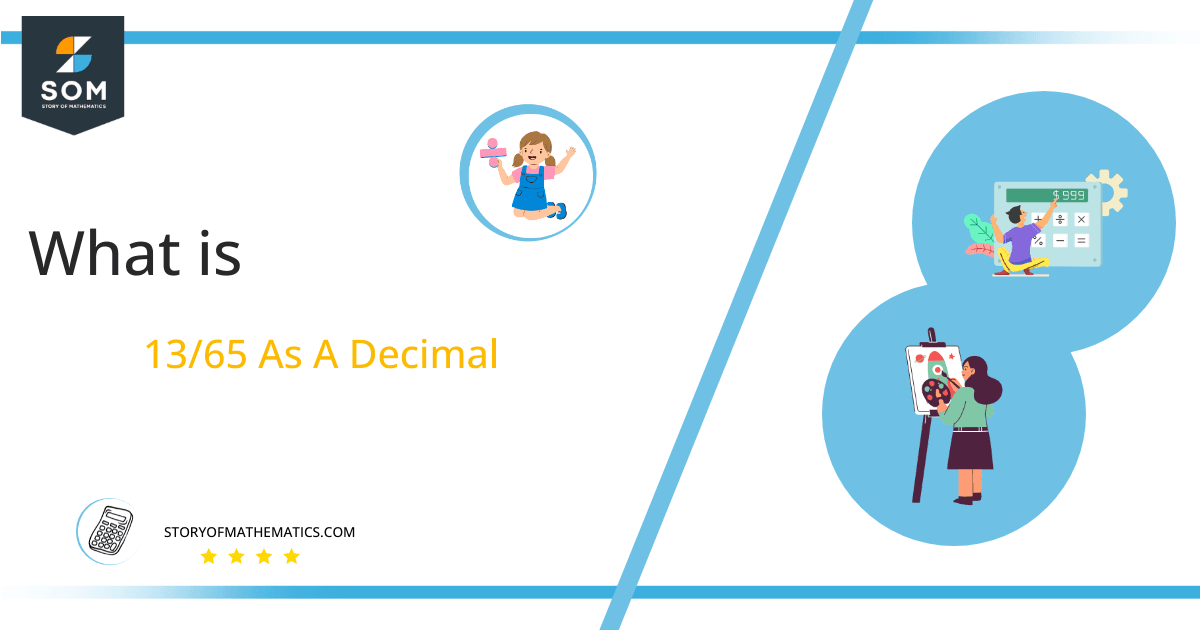# What Is 13/65 as a Decimal + Solution With Free Steps

The fraction 13/65 as a decimal is equal to 0.2.

Decimals, which are distinguished by the existence of a decimal point, are one of the key ideas in mathematics.  This decimal point is present between their fractional and whole number parts.  These are commonly generated as the solution of fractions.

Here, we are more interested in the division types that result in a Decimal value, as this can be expressed as a Fraction. We see fractions as a way of showing two numbers having the operation of Division between them that result in a value that lies between two Integers.Now, we introduce the method used to solve said fraction to decimal conversion, called Long Division, which we will discuss in detail moving forward. So, let’s go through the Solution of fraction 13/65.

## Solution

First, we convert the fraction components, i.e., the numerator and the denominator, and transform them into the division constituents, i.e., the Dividend and the Divisor, respectively.

This can be done as follows:

Dividend = 13

Divisor = 65

Now, we introduce the most important quantity in our division process: the Quotient. The value represents the Solution to our division and can be expressed as having the following relationship with the Division constituents:

Quotient = Dividend $\div$ Divisor = 13 $\div$ 65

This is when we go through the Long Division solution to our problem as shown in figure 1.Figure 1

## 13/65 Long Division Method

We start solving a problem using the Long Division Method by first taking apart the division’s components and comparing them. As we have 13 and 65, we can see how 13 is Smaller than 65, and to solve this division, we require that 13 be Bigger than 65.

This is done by multiplying the dividend by 10 and checking whether it is bigger than the divisor or not. If so, we calculate the Multiple of the divisor closest to the dividend and subtract it from the Dividend. This produces the Remainder, which we then use as the dividend later.

Now, we begin solving for our dividend 13, which after getting multiplied by 10 becomes 130.

We take this 130 and divide it by 65; this can be done as follows:

130 $\div$ 65 = 2

Where:

65 x 2 = 130

This will lead to the generation of a Remainder equal to 130 – 130 = 0.

Thus, we have a Quotient generated as 0.2=z, with a Remainder equal to 0.Images/mathematical drawings are created with GeoGebra.